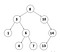# The Basics of Graphs and Trees

Graphs and trees are central to the concept of data structures in computer science and represent a large category within the subject, among others like lists (arrays) and hashes. They are something many to-be employees at tech firms need to know well. Many interviewees find tree and graph questions to be some of the hardest problems to solve. Due to the nature of their structure it is trickier to traverse as opposed to a linear structure like an array or hash.

# Trees

A tree is a structure comprised of nodes, with each node having zero or more children nodes. Another key aspect of a tree is the root, which also has zero or more children. A tree can branch from a root node any number of ways, how many children it has and how many children the subsequent children nodes have will define the type of tree it is. For example a binary tree is one with two or less children for every node. Below would be an example of a binary tree up until the bottom left portion (subtree) where it is a ternary tree, so this would technically be disqualified as a binary tree and be defined as a general tree.

There are many different types of trees but there are a handful that serve as the main ones.

## Binary Tree

Defined as having no more than two children, a Binary Tree is probably the most popular and most common tree type you will find. With that there are extensions from this tree type that provide more restrictions than the dual node structure.

Binary Search Tree: is a binary tree in which the left child value of a node should be less than or equal to the parent value.Binary Search Tree (notice the value of the nodes on the respective sides)

This value property makes it primed for search operations since we can easily distinguish if the next node is in the left or right, hence the name Binary Search Tree.

AVL Tree: Within the same category of a binary search tree there is also an AVL tree, aptly named after its creators Adelson-Velshi and Landis. The primary factor discerning an AVL tree from a BST is the value of the balance factor, which should be less than or equal to 1. Unlike a BST an AVL has elements on both sides of the tree to be balanced. The balance factor equation is as follows:

`Balance Factor(BF) = height(left subtree) — height(right subtree)`

To balance itself an AVL will rotate nodes when unbalanced. A somewhat abstract idea but this attribute is how to maintain the AVL structure. At each node you check if the condition is met (BF ≤ 1)if so, move on to the next node, if not, perform a rotation to satisfy the condition. The type of unbalance will dictate the type of rotation, when a tree is said to be right unbalanced, you perform a left rotation.

## Why trees?

At this point you might be wondering what is the use of all of this? Well, trees are beneficial in many ways, any time we want to organize something in a hierarchical structure we want to use a tree. An example would be our computer’s file structure, we even use the term root for the base directory. It can allow for quicker search compared to a linked list but still slower than an array. Insertion and deletion is quicker than an array since we will not need to reorder every element after insertion(x).

# Graphs

A tree is actually a type of graph but the reverse is not always true; similar to the relationship of a rectangle and square. Like trees there are many different types of graphs but there are some main ones that will come up more commonly. Generally speaking graphs are made of Vertices and Edges. A single vertex is typically denoted as a circle or square with either an integer or letter (it can be anything but these two are the most common when speaking abstractly) representing its underlying value. An edge is a pair of vertices and is denoted with a line.

Adjacency is an important concept of graphs, using the above example, 6 is adjacent to 4 and can be denoted as (6,4) or 6 ~ 4, and in the case of an Undirected Graph the reverse is true, (4,6) or 4 ~ 6, we say that this relationship is symmetric. Using this same logic we could write the Edge set as {(4,6),(4,5),(3,4),(3,2),(2,5)),(1,2)),(1,5)}. Naturally there is also a Directed Graph, which does not share the same same property of an undirected graph in terms of adjacency. In order for something to be adjacent in a directed graph the edge set must be included, which is not necessarily true for an undirected graph since the reverse can be denoted which would include two edge sets.

Cyclic attributes are part of a graph structure as well, to breakdown the above into subgraphs, Edge set {(B,D),(D,B),(B,E),(E,D)} is set to be cyclical since they loop and are connected. Edge set {(B,D),(D,B),(B,C),(D,C)} would be acyclic with the endpoint being Vertex C. Directed Acyclic Graphs have their own category and are often called simply a “Dag”.

## Why graphs?

Graphs in computer science are often representations of networks. If you have ever seen a Verizon commercial in which they show the map of the USA with all the lines connected the cities, that is a real life example of an undirected graph is a typical of use for them. Roads and maps are also commonplace use cases for graphs. The World Wide Web itself is an example of a directed graph. Graphs make for an effective way to organize data points and relational data and offer an efficient way to access them.

sources:

Cracking the Coding Interview, by Gayle Laakmann McDowell

http://web.cecs.pdx.edu/~sheard/course/Cs163/Doc/Graphs.html

https://www.geeksforgeeks.org/graph-types-and-applications/